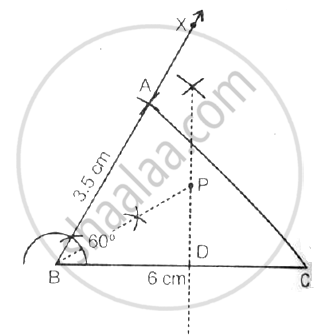# I. Construct  Abc, Where Ab = 3.5 Cm, Bc = 6 Cm and Abc = 60o. Ii. Construct the Locus of Points Inside the Triangle Which Are Equidistant from Ba and Bc. Iii. Construct the Locus of Points Inside the Triangle Which Are Equidistant from B and C. Iv. Mark the Point P Which is Equidistant from Ab, Bc and Also Equidistant from B and C. Measure and Records the Length of Pb. - Mathematics

Use ruler and compasses only for this question:

I. Construct  ABC, where AB = 3.5 cm, BC = 6 cm and ABC = 60o.
II. Construct the locus of points inside the triangle which are equidistant from BA and BC.
III. Construct the locus of points inside the triangle which are equidistant from B and C.
IV. Mark the point P which is equidistant from AB, BC and also equidistant from B and C. Measure and records the length of PB.

#### Solution

Steps of constructions:

1) Draw a line BC = 6 cm and CBX = 60°. Cut off AB = 3.5 cm. Join AC, ΔABC is the required triangle.

2) Draw perpendicular bisector of BC and bisector of ∠B.

3) The bisector of ∠B meets bisector of BC at P, therefore BP is the required length,
Where BP = 3.5 cm

4) P is the point which is equidistant from BA and BC, also equidistant from B and C.Concept: Constructions Under Loci
Is there an error in this question or solution?